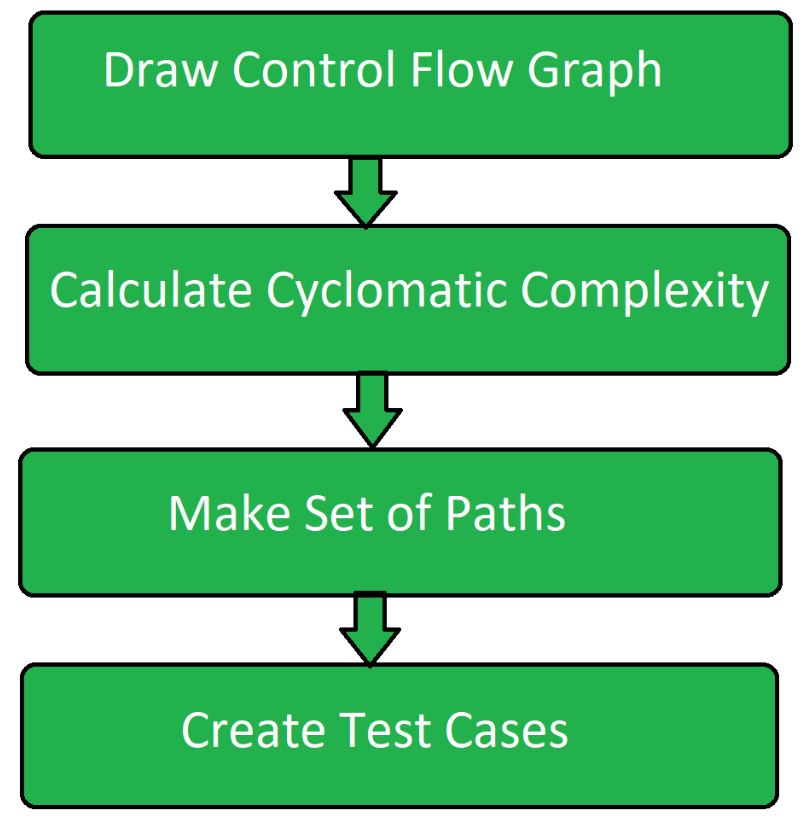# Path Testing in Software Engineering

• Last Updated : 02 Jul, 2020

Path Testing is a method that is used to design the test cases. In path testing method, the control flow graph of a program is designed to find a set of linearly independent paths of execution. In this method Cyclomatic Complexity is used to determine the number of linearly independent paths and then test cases are generated for each path.

It give complete branch coverage but achieves that without covering all possible paths of the control flow graph. McCabe’s Cyclomatic Complexity is used in path testing. It is a structural testing method that uses the source code of a program to find every possible executable path.

Path Testing Process:• Control Flow Graph:
Draw the corresponding control flow graph of the program in which all the executable paths are to be discovered.
• Cyclomatic Complexity:
After the generation of the control flow graph, calculate the cyclomatic complexity of the program using the following formula.
```McCabe's Cyclomatic Complexity = E - N + 2P

Where,
E = Number of edges in control flow graph
N = Number of vertices in control floe graph
P = Program factor ```
• Make Set:
Make a set of all the path according to the control floe graph and calculated cyclomatic complexity. The cardinality of set is equal to the calculated cyclomatic complexity.
• Create Test Cases:
Create test case for each path of the set obtained in above step.

Path Testing Techniques:

• Control Flow Graph:
The program is converted into control flow graph by representing the code into nodes and edges.
• Decision to Decision path:
The control flow graph can be broken into various Decision to Decision paths and then collapsed into individual nodes.
• Independent paths:
Independent path is a path through a Decision to Decision path graph which cannot be reproduced from other paths by other methods.